Open In App
Related Articles
• CBSE Class 9 Maths Revision Notes
• CBSE Class 9 Maths Formulas
• NCERT Solutions for Class 9 Maths
• RD Sharma Class 9 Solutions

# Real Numbers

Real numbers are continuous quantities that can represent a distance along a line, as Real numbers include both rational and irrational numbers. Rational numbers occupy the points at some finite distance and irrational numbers fill the gap between them, making them together to complete the real line. So, in other words, real numbers are those numbers that can be plotted on the real line.

The collection of all Rational numbers together is denoted by R and contains all the other numbers like natural numbers, integers, rational as well as irrational. Some examples of real numbers are 3 (a whole number), -1 (an integer), 1/2 (a rational number), √2 (an irrational number), π (an irrational number), 2.5 (a decimal number), etc.

In this article, you will learn everything about Real numbers like their properties, representation on a number line, decimal expansion, etc.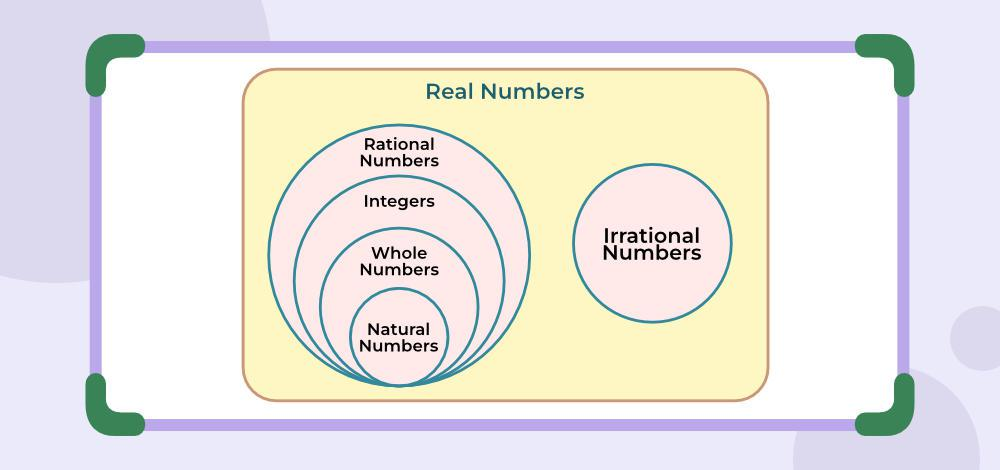## What are Real Numbers?

Ever thought that we can count things, but how can we count? We can count by the use of numbers. But the number is also of many types some have negative values, some have positive values, some are very large, some are very small, and some are in mathematical operations, so there are many types of numbers.

The number or the numeral system is the system of representing numbers. There are various types of number systems in maths like binary, decimal, etc. The Number system represents how a number should be written.

## Set of Real Numbers

All the numbers known to humans except the imaginary numbers come under the set of the real number. We use R to denote the set of real numbers. We can have various subsets of the real number that denote different types of numbers. Various subsets of the Real number are,

### Subsets of Real Numbers

Real Numbers can be divided into the following subsets:

• Natural Numbers
• Whole Numbers
• Integers
• Rational Numbers
• Irrational Numbers

Now let’s learn about these numbers in detail further in the article. The image added below shows various types of numbers.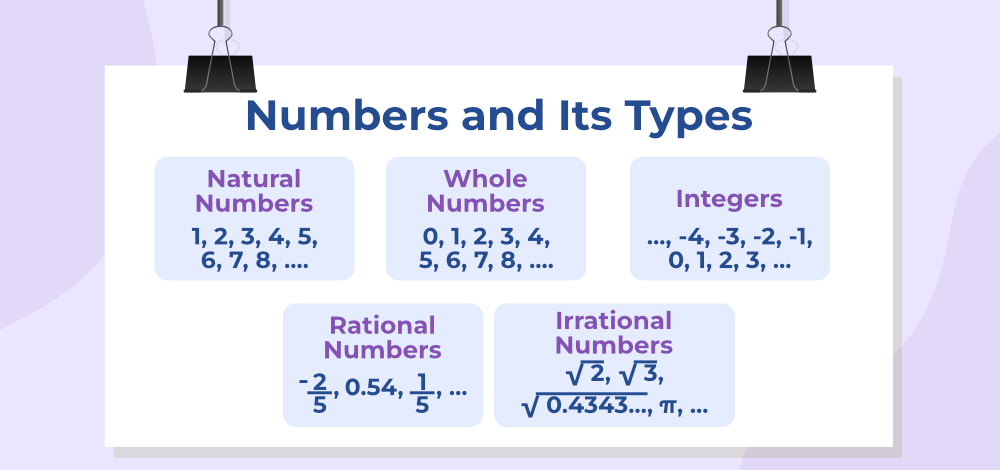### Natural Numbers

Natural numbers are the counting numbers i.e., numbers we use in our daily life for counting purposes, like 1, 2,3, 4, 5, and so on. As there can’t be -2 apples in the real world and also counting always starts with 1, not 0. So, only 1, 2, 3, 4, 5,… to infinity, are the only natural numbers, and these numbers are denoted by the symbol N

### Whole Numbers

Adding 0 to Natural Numbers makes the series a set of Whole Numbers. This collection of numbers {0, 1, 2, 3, 4, 5,…}, are known as whole numbers and denoted by the symbol W. The reason these numbers are called the whole number is that these numbers contain all the positive integers, which makes them complete, another name for complete is whole.

### Integers

The collection of the whole number and negative natural numbers together is called integers; thus, integers include positive numbers, negative numbers, and one neutral number i.e., 0. {…, -3, -2, -1, 0, 1, 2, 3,…} are a representation of integers in collection form, other than these integers are denoted by the symbol Z.

### Rational Numbers

Rational Numbers are numbers that can be expressed as the fraction p/q of two integers, a numerator p, and a non-zero denominator q such as 2/7. For example, 25 can be written as 25/1, so it’s a rational number. Some more examples of rational numbers are 22/7, 3/2, -11/13, -13/17, etc. As rational numbers cannot be listed in the collection form, the only representation of the rational numbers is Q.

### Irrational Numbers

Irrational numbers are those which cannot be expressed in the form of p/q where p and q are both integers and q ≠ 0. In short, irrational numbers are real numbers that are not rational numbers. √2 is an irrational number as it can’t be written in p/q form i.e., in √2/1, √2 is not an integer. √3, √5, π, etc. are some more examples of irrational numbers.

## List of Real Numbers

The list of real numbers is endless because it includes all kinds of numbers like whole, natural, integers, rational, and irrational numbers. Since it includes integers it has negative numbers too. So, there is no specific number from which the list of real numbers starts or ends. It goes to infinity towards both sides of the number line.

### Symbol of Real Numbers

We use R to represent a set of Real Numbers and other types of numbers can be represented using the symbol discussed below,

• N – Natural Numbers
• W – Whole Numbers
• Z – Integers
• Q – Rational Numbers
• Q’ – Irrational Numbers

## Real Numbers Chart

Rational Numbers, Irrational Numbers, and all the subsets of rational numbers all come under real numbers, and the real number chart is used to express all the branches of real numbers. The real number chart is added below: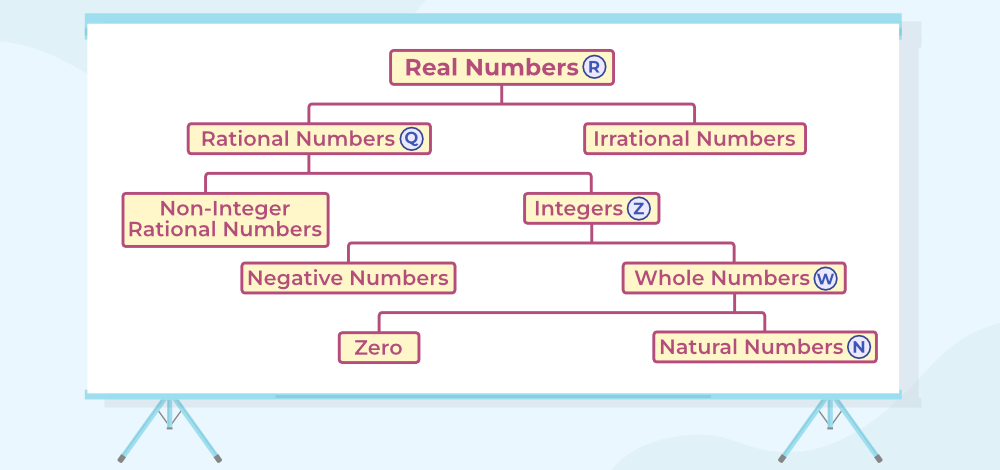## Properties of Real Numbers

There are different properties of Real numbers with respect to the operation of addition and multiplication, which are as  follows:

• Commutative Property
• Associative Property
• Distributive Property
• Identity Property
• Inverse Property

## Representation of Numbers on Number Line

A number line is a representation of Numbers with a fixed interval in between on a straight line. A Number line contains all the types of numbers like natural numbers, rational numbers, Integers, etc.

### Representation of Rational Numbers on the Number Line

As shown in the following number line 0 is present in the middle of the number line. Positive integers are written on the right side of zero whereas negative integers are written on the left side of zero, and there are all possible values in between these integers.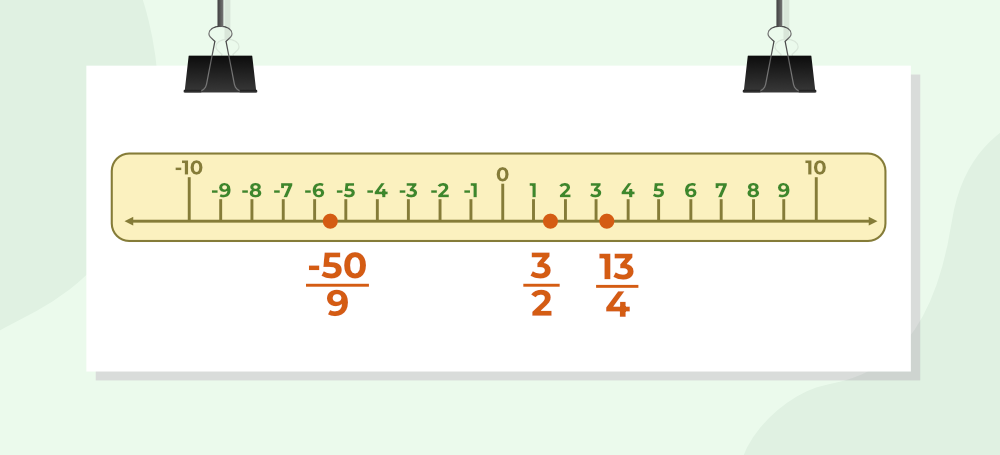Rational numbers are written between the numbers they lie. For example, 3/2 equals to 1.5, so is noted between 1 and 2. It shows that the number 3/2 lies somewhere between 1 and 2.

Similarly, the Number 13/4 = 3.25 lies between 3 and 4. So we noted it between 3 and 4. Number -50/9 = -5.555. . . , lies between -5 and -6. So we noted it between -5 and -6 on the number line.

Example: Represent the Following numbers on a number line:

• 23/5
• 6
• -33/7

Solution:

The rational numbers,

• 23/5
• 6
• -33/7

can easily be represented in a number line as,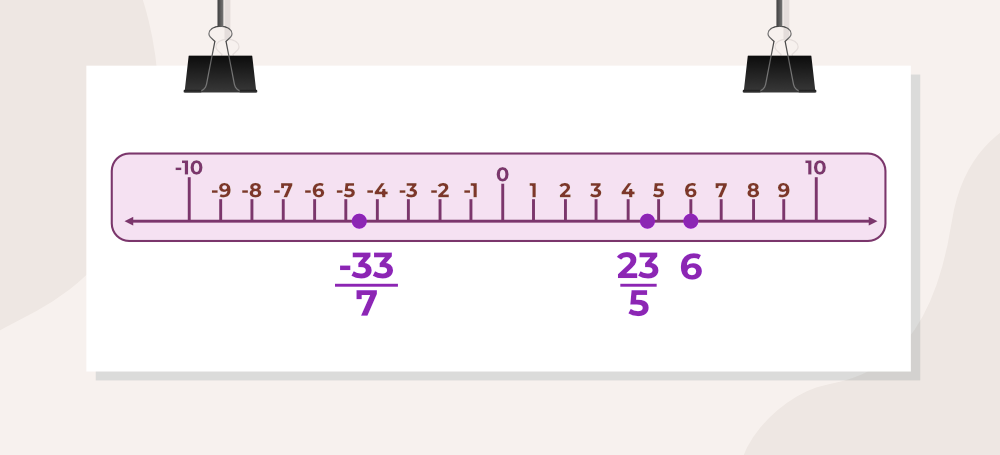### Representation of Irrational Numbers on Number Line

Irrational Numbers can’t be represented on the number line as it is, we need clever tricks and geometry to represent irrational numbers on a number line. Learn more about the Representation of √3 on a number line.

## Successive Magnification

The process of representation and visualization of real numbers on the number line through a magnifying glass is known as successive magnification.

Let’s take the example of 3.25.

In the first line, we see that 3.25 lies between 3 and 4. Now take it a step forward. Now we zoom in between 3 and 4 as represented in the second line. Here we found that 3.25 lies between 3.2 and 3.3. Now again, zoom in between 3.2 and 3.3 which is represented in the third line. Here we can see the required value of 3.25.

So, we represented 3.25 on the number line using successive magnification.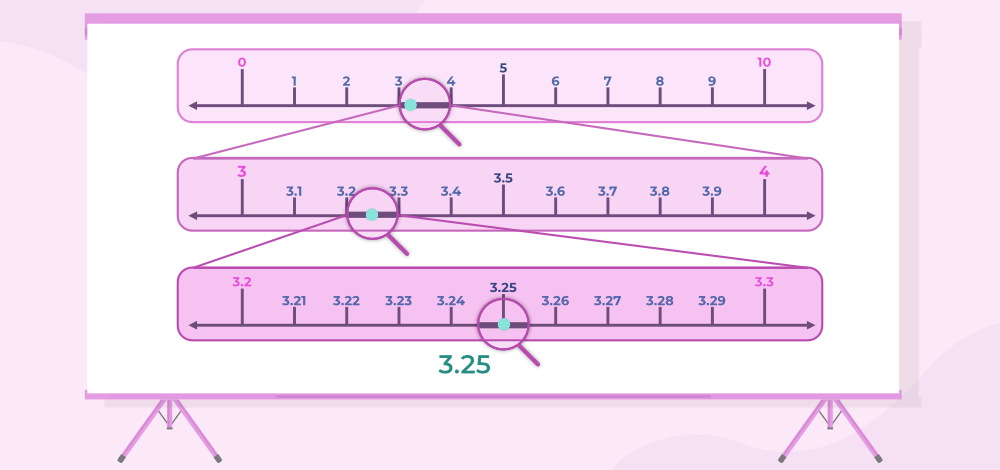## Decimal Expansion of Real Numbers

The decimal expansion of a real number is its representation in base equals to 10 (i.e., in the decimal system). In this system, each “decimal place” consists of a digit from 0 to 9. These digits are arranged such that each digit is multiplied by a power of 10, decreasing from left to right.

### Decimal Expansion of Rational Numbers

Can we represent 13/4 in another form that can show its exact value on the number line?

The answer is yes. We can write it in decimals which gives its exact value. Let’s Expand 13/4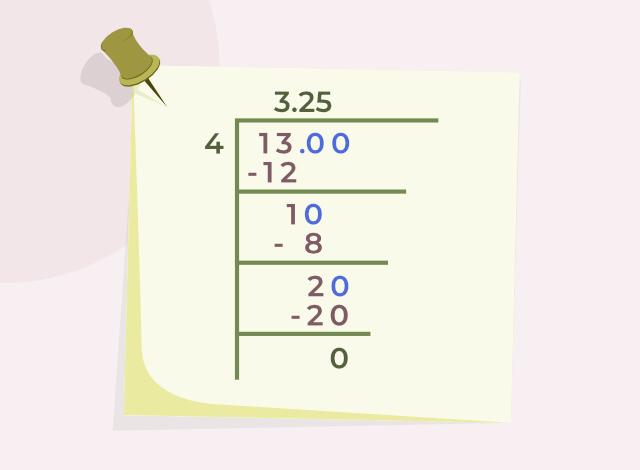So 13/4 can also be written as 3.25.

Now Let’s take another example. Let’s expand 1/3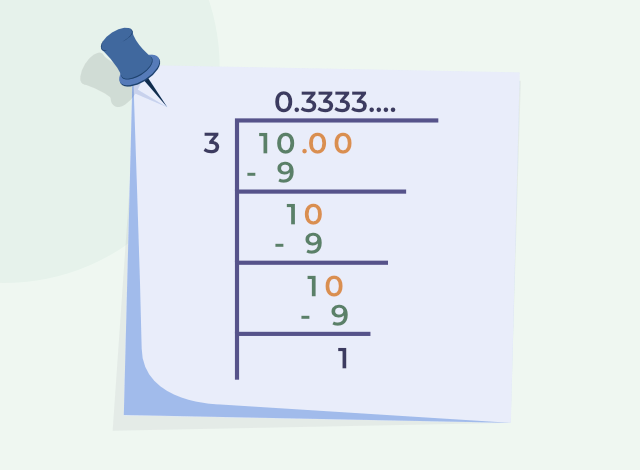So 1/3 can also be written as 0.3333…… We can also write it asSimilarly, 1/7 can be written as 0.142857142857142857… or. This is known as the recurring decimals expansion.

### Decimal Expansion of Irrational Numbers

Decimal Expansion of Irrational Numbers is non-terminating and non-repeating. We can find the decimal expansion such as √2, √3, √5, etc. using the long division method. The decimal Expansion of √2 is up to three digits after the decimal is calculated in the following illustration.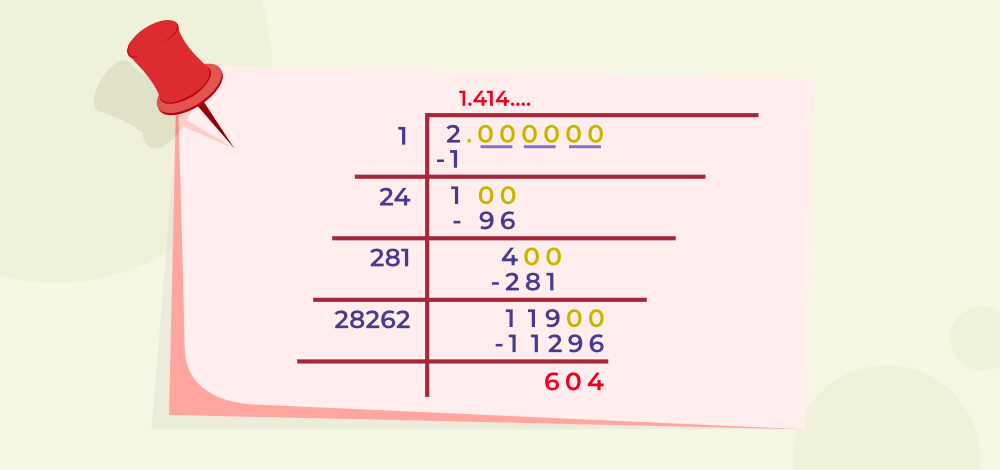## Operations on Real Numbers

We can perform mathematical operations, such as Division, Multiplication, Addition, and Subtraction, on real numbers and the result still be a real number. Also, on the subset of real numbers, such as Rational numbers, we can perform all the operations between two rational numbers and the result will always be rational numbers, but on Irrational numbers, when we perform mathematical operations, it can be either rational or irrational.

For example, the product of 3/5 and 5/7 (two rationals) is 3/7 which is a rational number but the product of √2 and 3√2 (two irrationals) is 6 which is rational.

## Sample Examples on Real Numbers

Example 1: Add √3 and √5

Solution:

(√3 + √5)

Now answer is an irrational number.

Example 2: Multiply √3 and √3.

Solution:

√3 × √3 = 3

Now answer is a rational number.

So we can say that result of mathematical operations on irrational numbers can be rational or irrational.

Now add a rational number with an irrational number.

Example 3: Add 2 and √5

Solution:

(2 + √5)

Now answer is an irrational number.

Example 4: Simplify the expression: (2 + √3)(5 + √3)

Solution:

(2 + √3)(5 + √3)

= 10 + 2√3 + 5√3 + 3

= 13 + 7√3

Which is an irrational number.

## FAQs on Real Numbers

### Q1: Define Real Numbers.

Collection of all rational as well as irrational numbers is called real numbers.

### Q2: What is the difference between a rational and an irrational number?

The key difference between rational and irrational number is rational numbers can be represented in the form of p/q, where p and q are integers and q≠0. Whereas irrational numbers can’t be represented in the same form.

### Q3: Are whole numbers and integers real numbers?

Yes, all whole numbers and integers are real numbers as whole numbers and integers are the subsets of the rational numbers.

### Q4: What is the symbol used to represent real numbers?

The symbol used to represent real numbers is OR R.

### Q5: What is a decimal representation of a real number?

Decimal Representation of a real number can be either terminating, non-terminating but repeating, or non-terminating non-repeating as a real number contains all real numbers as well as irrational numbers.